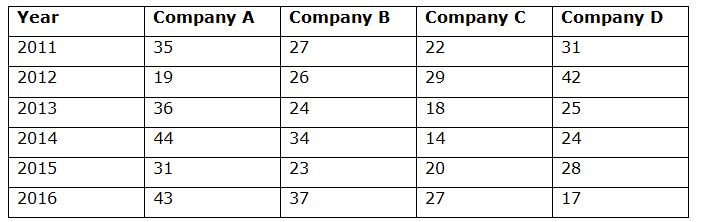# NIACL AO Prelims – Quantitative Aptitude Questions Day- 88

Dear Readers, Bank Exam Race for the Year 2019 is already started, To enrich your preparation here we have providing new series of Practice Questions on Quantitative Aptitude – Section. Candidates those who are preparing for NIACL AO Prelims 2019 Exams can practice these questions daily and make your preparation effective.

[WpProQuiz 5033]

Directions (1-5): What will come in place of the question mark (?) in the following questions?

1) (12 / 17) of 25% of 1020 = 120 +?

a) 60

b) 40

c) 80

d) 120

e) None of these

2) 25 × 26 ÷ √169 – 32 = ? – 42

a) 65

b) 57

c) 55

d) 67

e) None of these

3) 36% of 420 – 56% of 350 = ? – 94

a) 2

b) 2

c) -138.8

d) -158.8

e) None of these

4) (2/3) of 1(2/5) of 75% of 540 =?

a) 378

b) 756

c) 252

d) 332

e) None of these

5) (√9)3 × (√81)5 ÷ (27)2 = (3)(?)

a) 5

b) 4

c) 7

d) 6

e) None of these

Directions (6 – 10): Study the following table carefully and answer the given questions:

Following table shows the no of mobile phones (in lakhs) manufactured by four different companies in different years.6) Total no of mobile phones manufactured by Company C in all the given years is approximately what percentage more/less than the total no of mobile phones manufactured by Company B in all the given years?

a) 24 % less

b) 32 % more

c) 32 % less

d) 24 % more

e) None of these

7) If in the year 2017, total manufacturing of Company A, B, C and D be increased by 12 %, 14 %, 18 % and 21 % while comparing with the previous year respectively, then find the total manufacturing of all the given companies together in the year 2017?

a) 13452000

b) 15783000

c) 16894000

d) 14277000

e) None of these

8) Find the ratio between the total manufacturing of company A in the year 2012, 2014, 2015 and 2016 together and to that of Company D in the year 2011, 2013, 2015 and 2016 together?

a) 137 : 101

b) 125 : 98

c) 113 : 87

d) 102 : 75

e) None of these

9) The average no of mobile phones manufactured by all the given companies in the year 2013 is approximately what percentage of the average no of mobile phones manufactured by all the given companies in the year 2016?

a) 74 %

b) 83 %

c) 92 %

d) 107 %

e) 94 %

10) Find the difference between the total no of mobile phones manufactured by all the given companies in the year 2011 and 2012 to that of total no of mobile phones manufactured by all the given companies in the year 2014 and 2015 together?

a) 16 lakhs

b) 21 lakhs

c) 13 lakhs

d) 23 lakhs

e) None of these

Directions (1-5):

(12/17) × (25/100) × 1020 = 120 + ?

=> 180 = 120 + ?

=> ? = 180 – 120 = 60

[(25 × 26)/13] – 9 = ? – 16

=> 41 = ? – 16

=> ? = 41 + 16 = 57

[(420 × 36) / 100] – [(350 × 56) / 100]= ? – 94

=> 151.2 – 196 = ? – 94

=> ? = 151.2 + 94 – 196 = 49.2

(2/3) of 1(2/5) of 75% of 540 =?

? = 540 × (75/100) × (7/5) × (2/3) = 378

33 × (9)5 ÷ (33)2= 3?

=> 33 × 32 * 5 ÷  36 = 3?

=> 33+10-6 = 3?

=> 37 = 3?

=> ? = 7

Directions (6-10):

Total no of mobile phones manufactured by Company C in all the given years

= > 22 + 29 + 18 + 14 + 20 + 27

= > 130 lakhs

Total no of mobile phones manufactured by Company B in all the given years

= > 27 + 26 + 24 + 34 + 23 + 37

= > 171 lakhs

Required % = [(171- 130)/171]*100 = 23.97 % = 24 % less

In the year 2017, total manufacturing of Company A, B, C and D,

= > 43*(112/100) + 37*(114/100) + 27*(118/100) + 17*(121/100)

= > 48.16 + 42.18 + 31.86 + 20.57

= > 142.77 lakhs = 14277000

The total manufacturing of company A in the year 2012, 2014, 2015 and 2016

= > 19 + 44 + 31 + 43

= > 137 lakhs

The total manufacturing of company D in the year 2011, 2013, 2015 and 2016

= > 31 + 25 + 28 + 17

= > 101 lakhs

Required ratio = 137: 101

The average no of mobile phones manufactured by all the given companies in the year 2013

= > (36 + 24 + 18 + 25)/4

= > (103/4) lakhs

The average no of mobile phones manufactured by all the given companies in the year 2016

= > (43 + 37 + 27 + 17)/4

= > (124/4) lakhs

Required % = [(103/4)/ (124/4)]*100 = 83.06 % = 83 %

The total no of mobile phones manufactured by all the given companies in the year 2011 and 2012

= > 35 + 27 + 22 + 31 + 19 + 26 + 29 + 42 = 231 lakhs

The total no of mobile phones manufactured by all the given companies in the year 2014 and 2015

= > 44 + 34 + 14 + 24 + 31 + 23 + 20 + 28 = 218 lakhs

Required difference = 231 – 218 = 13 lakhs